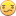## Simple Standard Deviaton

Hi there.

I have a simple problem that I just cant seem to find the answer to.

I have a list of observations with two of the variables being AccountNumber and TransactionAmount, there are mutliple transactions for one account.

Im trying to find the standard deviaton per account.

i currently have the following:-

proc means data=OutboundPayments std ;
class AccountNumber ;
var TransactionAmount ;
output sum=TransactionAmount out=Count_Amount ;

run;

while the results window does contain the correct standard deviation the output dataset "Count_Amount" does not and im struggling as to why.

Any help greatly appreciated.

1 ACCEPTED SOLUTION

Accepted Solutions

## Re: Simple Standard Deviaton

You only requested SUM on the output statement.

Try

output sum=TransactionAmountSum std=TransactionAmountSTD out=Count_Amount ;

4 REPLIES 4

## Re: Simple Standard Deviaton

In your OUTPUT statement you've only specified a SUM. Add STD to the list.art297
Opal | Level 21

## Re: Simple Standard Deviaton

You asked to output the sum rather than the standard deviation (std). Also, unless you want the overall std, include the nway option. e.g.:

```proc means data=sashelp.class std nway;
class sex ;
var height ;
output std=TransactionAmount out=Count_Amount ;
run;
```

Art, CEO, AnalystFinder.com

## Re: Simple Standard Deviaton

You only requested SUM on the output statement.

Try

output sum=TransactionAmountSum std=TransactionAmountSTD out=Count_Amount ;

## Re: Simple Standard Deviaton

Thank you for everyones replys on this.

So SimpleDiscussion stats
• 4 replies
• 690 views
• 4 likes
• 4 in conversation